Mobile QR Code1. (Dept. Mechatronics Convergence Engineering, Changwon National University, Changwon, 51140, Korea)

Particle method, Particle interaction model, Solid particle model, Mobile sea-bed behavior

## 1. Introduction

The ocean waves may not affect the sea bed due to energy dissipation owing to the distance between the free surface and the sea bed. However, when the water depth is shallow enough, or the energy of the waves is large enough (i.e. with a tsunami), the sea bed can be disturbed. The behavior of the sea bed may affect environmental and ocean structures, and thus, accurate estimation is important.

The breaking wave problems have been investigated theoretically, experimentally, and numerically (1,2) considering soil movement induced by breaking wave energy. Large deformation of the sea bed induced by tsunami waves was also studied (3). Despite numerous efforts to simulate sea bed motion due to waves, its complexity has dramatically increased, and has thus been restricted to the conventional numerical method.

With the conventional numerical method in the field of computational fluid dynamics, the grid system following the Eulerian approach is the most representative method. The Eulerian approach uses a grid as a control volume in fluid dynamics, and then expects physical quantities by measuring the in-and-out flow through the control surface. It is, indeed, a very efficient method to estimate fluid dynamics; however, its original concept of a grid system may cause numerical errors, which is a concern for accuracy.

The particle method follows the Lagrangian approach, and thus, all physical quantities can be calculated by tracing each particle. For the particle method, the Smoothed Particle Hydrodynamics (SPH) and Moving Particle Semi-implicit (MPS) methods are well-known. The SPH method was first announced in (4). At that time, the pressure on each particle was predicted by using the equation-of-state, and thus, pressure fluctuation was the most common issue with SPH. In (5), the authors used a Poisson pressure equation (PPE) for pressure calculation following the MPS method to suppress pressure fluctuation. The MPS method was originally proposed for incompressible flow (6). At that time, the MPS method focused on the impact load from the sloshing problem and the broken dam problem, and the PPE was adopted to predict loads induced by fluids. However, there was still pressure disturbance, and thus, a multi-source term for the PPE was investigated (7). Furthermore, the particle method can be achieved by tracking each particle, and the free surface searching model was very important. The free-surface searching model in (6) was modified in (8) by using multiple criteria to judge the free-surface particle. Another investigation suppressed the pressure perturbation by using a corrective matrix on the PPE equation (9).

The particle method has the advantage in a multi-phase flow. In (10), the authors studied multi-phase interaction models for the MPS method to reproduce the three-phase sloshing problem. In (11), the authors extended the MPS method to solid particles with a newly adopted solid particle interaction model to simulate the gravel dumping problem. In that investigation, the angle of repose on dumped solid particles was observed. Furthermore, the MPS method was coupled with the Direct Element Method (DEM) to simulate the sediment transport problem (12).

In this study, the newly developed MPS method simulated the mobile sea bed–behavior problem with large waves induced by a broken dam. The numerical results were compared with corresponding experimental results, and then, various densities of soil particles were investigated.

## 2. Moving Particle Semi-implicit Method

The MPS method is a computational fluid dynamics method following the fully Lagrangian approach. Its governing equations are continuity and Navier-Stokes’ equations, as follows:

D ρ D t = 0

##### (2)
D u D t = 1 ρ P + ν 2 u + g

where $\rho$ is density of the fluid, $t$ is time, $\overset{\rightarrow }{u}$ is velocity of a particle, $P$ is pressure on the particle, $\nu$ is a dynamic viscosity coefficient, and $\overset{\rightarrow }{g}$ is gravitational acceleration. Since the MPS method follows the particle method, all differential equations should be replaced by forms of the particle method, as follows:

##### (3)
ϕ i = d n 0 j i ϕ i j r i j | r i j | 2 r i j w | r i j |

##### (4)
ϕ i = d n 0 j i ϕ i j r i j | r i j | 2 w | r i j |

##### (5)
2 ϕ i = 2 d λ n 0 j i ϕ i j w | r i j |

where $\nabla$ is gradient, $\phi$ is arbitrary physical quantities, $d$ is the number of dimensions, $n_{0}$ is the particle number density in the initial arrangement, $w$ is a kernel function, $\lambda$ is an adjustment parameter, subscript $i$ denotes the i-th particle, and $\phi _{ij}$ denotes $\phi _{j}-\phi _{i}$. The adjustment parameter makes a value equal to an analytical solution, which can be calculated as follows:

##### (6)
λ = j i | r i j | 2 w | r i j | j i w | r i j |

The particle number density and kernel function are key parameters of the MPS method to keep the continuum of the system to avoid violating the laws of physics. The particle number density uses fluid density, and the kernel function measures the effect of neighboring particles on center particles. The particle number density and kernel function can be expressed as follows:

##### (7)
n i = j i w | r i j |

##### (8)
w | r i j | = 1 | r i j | r e 3 1 + | r i j | r e 3 0 r i j r e 0 r e r i j

According to Eq. (8), when the distance between center and neighboring particles exceeds the effective range ($r_{e}$), the kernel function has a value of zero, which means neighboring particles beyond the range cannot affect center particles. The effective range is set to $2.1l_{0}$ from a numerical convergence test, and $l_{0}$ is the particle distance in the initial arrangement.

For the solid particle problem, the viscosity term, which is the second term on the right-hand side of Eq. (2), needs to be eliminated. And then, the friction and drag terms will be replaced by a viscosity term. Thus, the governing equation of the MPS method for solid particles will be expressed as follows:

##### (9)
D u D t = 1 ρ P + 1 ρ F f t + F d t + g

where $\overset{\rightarrow }{F}_{f}$ is friction force and $\overset{\rightarrow }{F}_{d}$ is drag force.

The friction term consists of two forces; the friction force from the relative acceleration between the center and neighboring particles, and the accumulated weight from the center and neighboring particles over the center particle. Fig. 1shows a schematic of the friction force calculation.

The friction force due to relative acceleration can be calculated as follows:

##### (10)
F f 1 = j i 1 2 μ j + μ i ρ j a n , j ρ i a n , i t i j

##### Fig. 1. Schematic for friction force.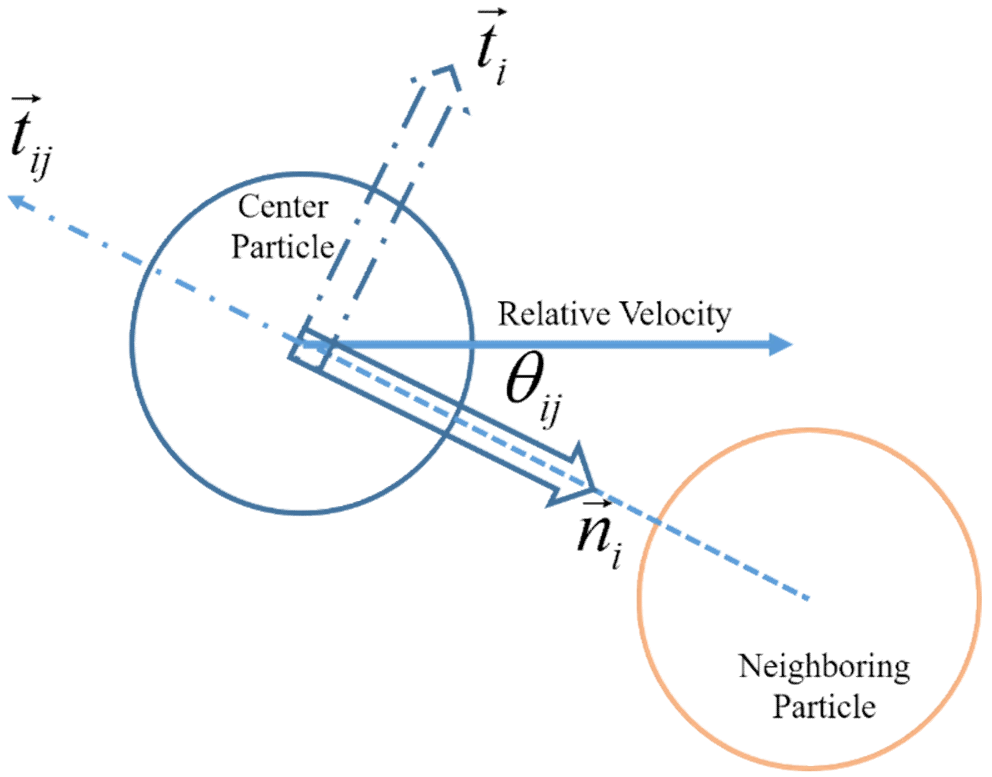##### Fig. 2. Schematic for accumulated weight.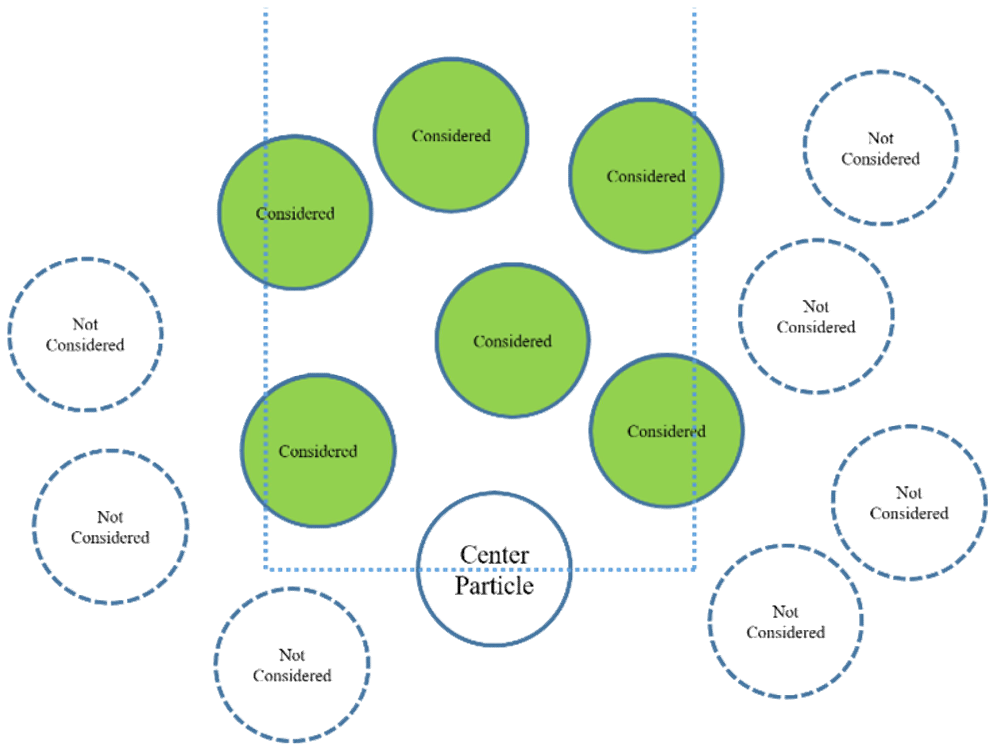##### Fig. 3. Schematic of the drag force calculation.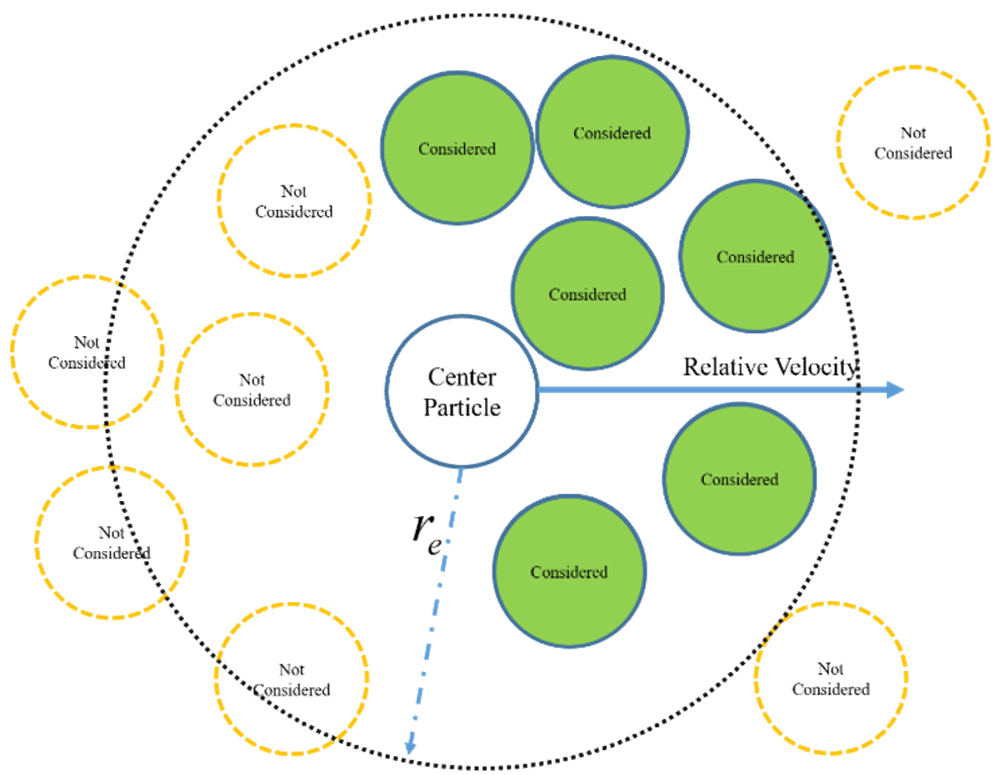where $\mu$ is a friction coefficient, $\overset{\rightarrow }{a}_{n}$ is a normal component of acceleration, and $\overset{\rightarrow }{t}$ is the unit tangential vector of the friction force.

Another term in friction force is accumulated weight friction force, which can be expressed as follows:

##### (11)
F f 2 = j i 1 2 μ j + μ i p s , i j sin θ i j t i j

where $\theta _{ij}$ is the angle between the connecting line and the moving direction of the center particle, and $p_{s,ij}$ is the accumulated weight of a stacked particle (see Fig. 2). The accumulated stacked particle weight can be calculated as follows:

##### (12)
p s , i j = p h , j + p h , i 2

where

##### (13)
p h , i = j i ρ j g     i f    o n l y    z j > z i    a n d    x i r e l 0 < x j < x i

The friction force can be calculated as the summation of the two terms above:

##### (14)
F f = F f 1 + F f 2         = 1 2 μ j + μ i j i ρ j a n , j ρ i a m , i + p s , i j sin θ i j t i j

For the calculation of drag force, the following equation was implemented:

##### (15)
F D = 1 r e 2 j i 1 2 C D n i l 0 2 v i j 2 w | r i j |

where $C_{D}$ is the drag coefficient, and $v_{ij}$ is the relative velocity between center and neighboring particles. In this case, the center particle should be a solid particle, and the considered neighboring particles are fluid particles only (see Fig. 3).

## 3. Simulation Results

Sea-bed behavior under violent waves was simulated for this paper. A model is shown in Fig. 4. Water was captured by a wall and the soil particles lay over the wall. A total of 144,000 particles were used for this simulation: 70,000 were water particles, and 52,000 were soil particles. The time interval for the simulation was 0.0001s, and the total simulation time was set to 1.5s in real time. The density of water was $1,000kg/m^{3}$, and the soil particles were $900kg/m^{3}$, the same as in the corresponding experiment.

When the wall moves upward at 0.5m/s, the captured water collapses spontaneously from gravitational acceleration. The collapsed water collides with the soil particles and disturbs them.

In the experiment, a polyethylene oxide (PEO) solution was used for the soil bed. The reason for using the PEO solution in the experiment was because the soil bed can be regarded as a liquid due to liquefaction effects. For this reason, fluid-soil and solid-soil sea beds were both simulated and compared.

##### Fig. 4. A model of the numerical simulation.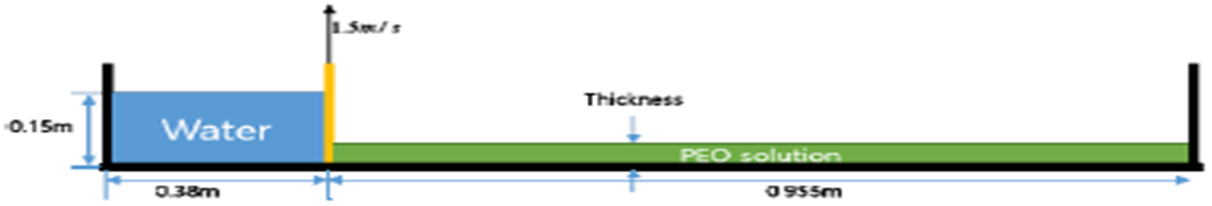##### Fig. 5. Comparison of snapshots from the experiment and the numerical simulation.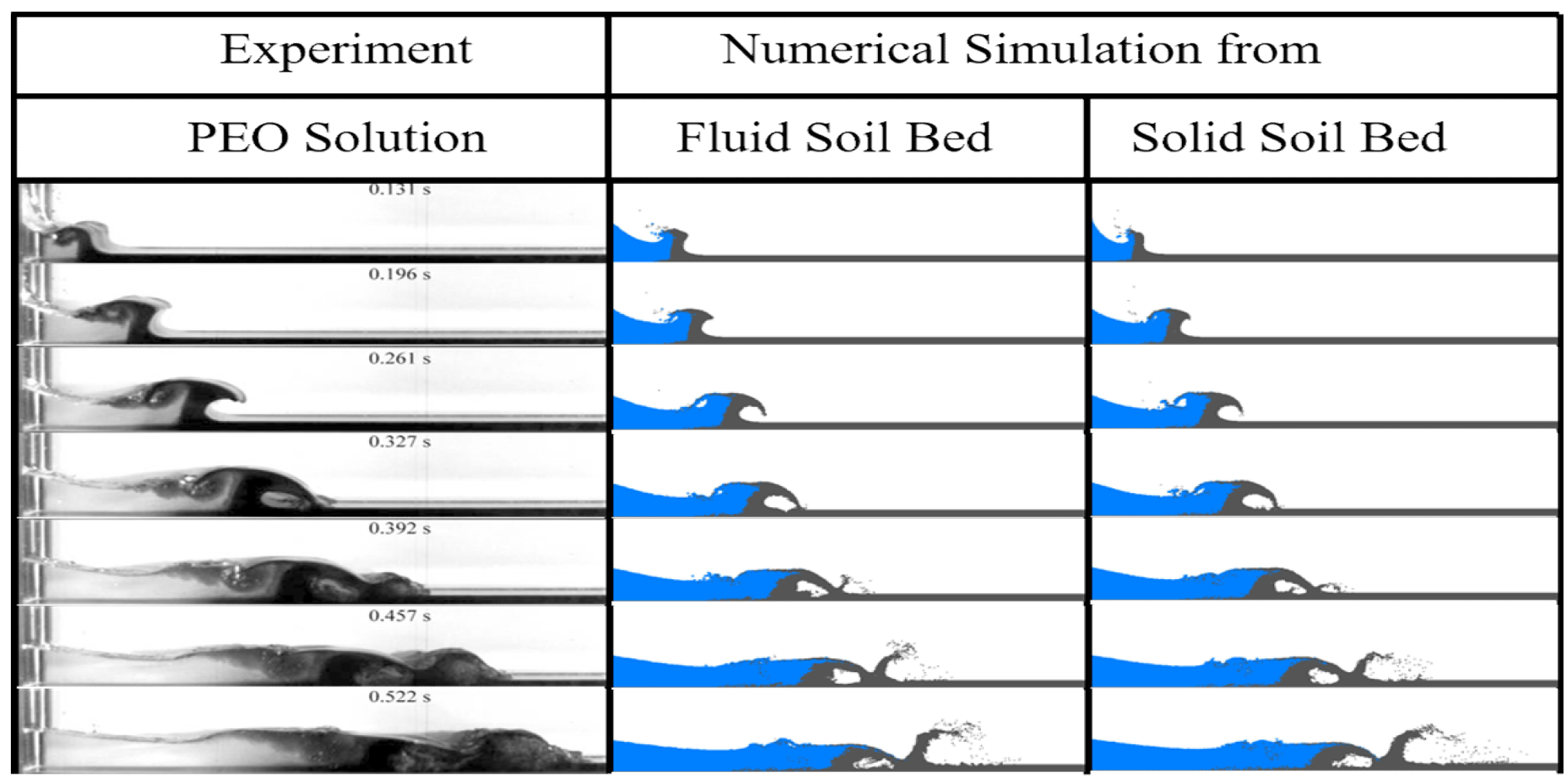##### Fig. 6. Comparison of pressure on the liquid soil particles (left) and on the solid soil particles (right).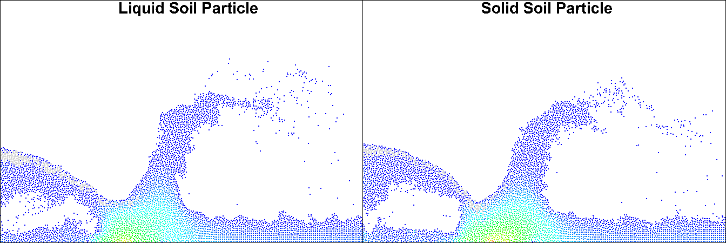Fig. 5shows snapshots from the experiment and both of the numerical simulations of the fluid soil bed and the solid soil bed over an arbitrary time period. When the gate opened, the captured water column collapsed spontaneously from gravitational acceleration. The collapsed water collided with the soil bed beneath the soil layer. The water scooped up the soil bed, and the wave energy transferred into the soil particles. The disturbed soil particles had the shape of a wave, and it propagated to the right end of the tank. The soil bed wave also had a leading edge, the same as the water wave, creating an overturning wave. The leading edge of the overturning wave made a rolling shape that scooped up the soil bed layer.

Figs. 6 and 7 represent a detailed comparison between the numerical results from the MPS method for fluid and solid particle velocity. In Fig. 6, the soil particle waves are compared. The contour of the particles denotes the pressure. From this comparison, the pressure on the particles and the tendency of the generated waves were not different. The velocity vector on the soil particles is visualized in Fig. 7. As mentioned with Fig. 6, the difference was too small to specify; however, a comparison of velocities on the particles shows a significant difference. The liquid soil particle movement has the form of a splash, and thus, the velocity tendency looks like hydraulic jumping. Another point with the liquid model is that the collision model in the algorithm affects particle movement to add velocity. With the solid soil particles, the soil bed wave was generated in a way similar to the liquid soil bed; however, the velocity tended to have a lateral direction due to the pushing effects of the soil bed, and the collision model did not influence the particles. In this regard, the velocity of solid particles after the collision is smaller, compared to liquid particles.

##### Fig. 7. Comparison of velocity on the liquid soil particles (left) and the solid soil particles (right).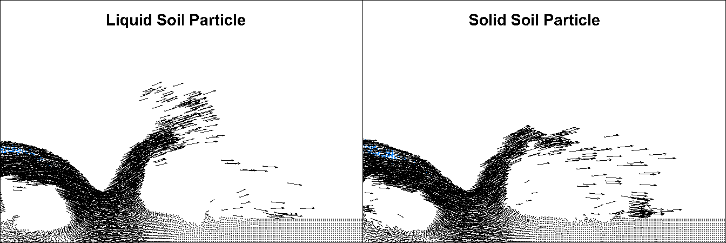The wave-like soil bed movement was also observed in both the numerical simulation for the liquid MPS method and the solid MPS method. However, a few differences were observed. The prior point of difference was the shape of the wave edge of the soil particle. With liquid soil, the soil particle looks like it is being pushed, but the result from the solid soil bed was a carpet-shaped wave edge, and it was more similar to the experiment. Another difference was the development of a soil bed wave. When the overturned soil particle wave stroke the soil bed, the liquid particle was exaggerated, compared to the solid particle. In the MPS method, there is a collision model that is made to avoid unexpected particle concentrations and to calculate collision effects. It is one of most important factors in simulating fluid dynamics for the particle method; however, it might not be useful for a solid particle method. The spreading shape of the soil particles after the soil bed collision also differed. The solid particles splashed further than in the liquid model. This is due to the viscosity effects on the liquid model, which might not be considered.

## 4. Conclusion

In this study, the MPS method was used to simulate mobile-bed behavior under a large-wave collision problem. To demonstrate soil particle behavior, the MPS method for liquid particles was modified and developed. For modification and development, friction- and drag-force models were implemented instead of a viscosity model.

For validation of the accuracy of a solid particle program, the results from the MPS method for solid particles was compared to the MPS method for liquid particles. Both numerical simulation results were compared to corresponding experimental results. The numerical simulations agreed well with the experimental results; however, differences were observed between the MPS method for the liquid model and the solid model. For a macro view of the comparison, the tendency of the soil bed behavior was similar; however, the details were dissimilar. The MPS for the solid model had a more blunt leading edge in the soil particle wave, and smoother behavior on soil wave propagation.

The MPS method has been extended in its applicability to not only the fluid dynamics problem, but also to the solid particle problem, including the fluid\textendash{}solid interaction problem. It would be useful to study a more complicated problem, such as the scour and erosion problem, in the future.

### ACKNOWLEDGMENTS

This research was supported by the National Research Foundation of Korea (NRF) funded by the Ministry of Education (NRF-2018R1D1A1B07048254).

### REFERENCES

1
Chenson H., 2009, Application of the method of characteristics to the dam break wave problem, J. Hydraul. Res., Vol. 47, pp. 41-492
Liao C. B., Wu M. S., Liang S. J., 2009, Numerical simulation of a dam break for an actual river terrain environment, Hydrol. Process, Vol. 21, pp. 447-4603
Janosi I. M., Jan D., Szabo K. G., 2004, Turbulent drag reduction in dam break flows, Exp. Fluids, Vol. 37, pp. 219-2294
Monaghan J.J., 1994, simulating free surface slows with SPH, J. Comput. Phys., Vol. 110, No. 2, pp. 39-4065
Xu R., Stansby P., Laurence D. L., 2009, Accuracy and Stability in incompressible SPH based on the projection method and a new approach, J. Comput. Phys., Vol. 228, No. 18, pp. 6703-67256
Koshizuka S., Oka Y., 1996, moving particle semi-implicit method for fragmentation of incompressible fluid, Num. sci. and Eng., Vol. 123, pp. 421-4347
Tanaka M., Masunaga T., 2010, Stabilization and smoothing of pressure in MPS method by quasi-compressibility, J. Comput. Phys., Vol. 229, No. 11, pp. 4279-42908
Lee B.H., Park J.C., Kim M.H., 2010, Numerical simulation of impact loads using a particle method, Ocean Eng., Vol. 37, pp. 164-1739
Duan G., Matsunaga T., Yamaji A., Koshizuka S., Sakai M., 2020, Imposing accurate wall boundary conditions in corrective-matrix-based moving particle semi-implicit method for free surface flow, Int. J. Numerical method in fluids10
Kim K.S., Kim M.H., 2015, Simulation of Multiliquid-layer sloshing with vessel motion by using moving particle semi-implicit method, J. offshore Mech. And Arctic Eng., Vol. 137, 051602-111
Kim K.S., Kim M.H., Jang H., Cho H.C., 2018, Simulation of solid particle interactions including segregated lamination by using MPS method, Comp. Modeling of Eng. Sci., Vol. 116, No. 1, pp. 11-2912
Harada E., Gotoh H., Ikari H., Khayyer A., 2019, Numerical simulation for sediment transport using MPS-DEM coupling model, Advanced in Water Resource, Vol. 129, pp. 354-364## Author

Kyung Sung Kim is an Associate Professor of Dept. Mechatronics Convergence Engineering at Changwon National University, Changwon, Korea. He received a bachelor degree and a master’s degree in Naval Architecture and Ocean Engineering from Pusan National University in Korea. He then moved to Texas in the U.S.A., and earned a PhD in Ocean Engineering from Texas A&M University. He was employed at the Pohang University of Science and Technology as a Research Professor. His major field is hydrodynamics and computational fluid dynamics, including particle methods. However, he is now in charge of experimental approaches to offshore experiments for education. He is working to develop efficient methods of educating young researchers with fourth industrial revolution technology in both numerical and experimental research.